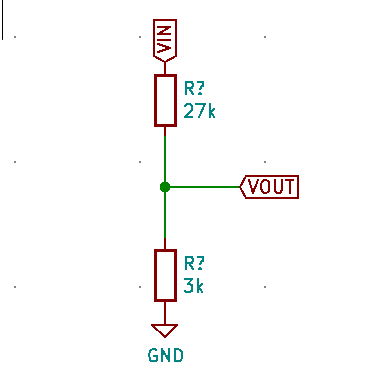# Daily Log 2020-11-19

2020-11-19

## Stepping down a large voltage for sensing

Typically I would use a simple voltage divider for this.When using the Analog to Digital Converter (ADC) on the ATtiny85 this poses a problem because in order to keep the power loss to a minimum the impedance of the circuit will exceed the maximum input impedence.

### Example:

I'm expecting an input voltage between 0 and 50V, the ADC can read from 0 to 5V so I want to step down the input by a factor of 1/10th. The formula for a voltage divider is `Vout = (R2 / (R1 + R2)) * Vin`. Taking an R1 = 90Ω and R2 = 10Ω we get the correct output voltage of 0 to 5V. However it will come at enormous cost to power. We can compute the current so at the peak of 50V :

``````
I = V / R
P = I * V
P = V^2 / R

V = 50V
R = 100Ω
P = 2500 / 100Ω = 25W
``````

25 Watts is a HUGE amount of power, to adjust that we can increase the resistance of the resistors to offset that. Using `R1 = 27kΩ, R2 = 3kΩ` we preserve the 1/10 ratio and reduce the power consumption to 1.8 mA. This would seem to solve our problem except the ADC also has a maximum input impedance of 10kΩ. Running through our equations again:

``````
V = 50V
R = 30kΩ
P = 2500 / 30000 = 83mW
``````

### Solution:

A solution to this problem is to use an OpAmp as a buffer. An OpAmp with its output wired to it's input works as a 1:1 amplifier (aka a buffer) but has the useful properties of isolating the input and output sides. An OpAmp has a "near infinite" input impedance which prevents current leakage from the 50V inputs, but a near zero output impedance, which allows our ADC to sample the outputs within its maximum allowable input impedance (10kΩ).## I2C

In the past I've found the Arduino ecosystem difficult to work in; I want to use my own editor, manage my libraries myself, and more control over compilation, linking, and programming. When I set out to work on the ATtiny85 I decided I'd do it outside of the Arduino ecosystem but that choice brought with it some challenges when trying to find good open source libraries for common requirements like I2C. After spending a couple of days trialling a number of I2C options I ended up writing my own based on existing sources.

## Libraries I looked into

• TinyWire - This library worked without Arduino but took up over a kilobyte of rom (nearly 25% of the available storage space for the ATtiny85).
• ATTinyCore - This is an arduino core that comes with a ton of useful functionality including I2C libraries. Unforunatly it seems very baked into the Arduino ecosystem which I was hoping to avoid.
• tiny-i2c - This is my favorite so far. It's small (compiles down to less than 400bytes) but depends on Arduino headers. When I vendored in the Arduino headers it compiled but wasn't working.

You can find my attempt at meeting the requirements for my project at my twi github project.